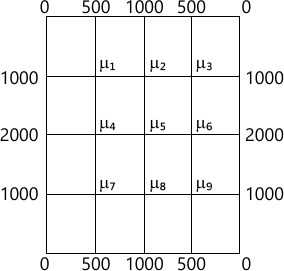MORE IN Engineering Mathematics 3
VTU Computer Science (Semester 3)
Engineering Mathematics 3
June 2013
Total marks: --
Total time: --
INSTRUCTIONS
(1) Assume appropriate data and state your reasons
(2) Marks are given to the right of every question
(3) Draw neat diagrams wherever necessary

1 (a) Obtain the Fourier series expansion of $f(x)=\left\{\begin{matrix} x,&if &0\le x \le \pi \\2\pi -x, &\if &\pi \le x \le 2\pi \end{matrix}\right.$ and hence deduce that $\dfrac {\pi^2}{8}=\dfrac {1}{1^2}+\dfrac {1}{3^2}+\dfrac {1}{5^2}+......$
7 M
1 (b) Find the half range Fourier sine series of $f(x)= \left\{\begin{matrix}x, &if &0 6 M 1 (c) Obtain the constant term and coefficients of first cosine and sine term in the expansion of y from the following table:  x 0 60° 120° 180° 240° 300<°/td> 360° y 7.9 7.2 3.6 0.5 0.9 6.8 7.9 7 M 2 (a) Find the Fourier transform of \[ f(x)=\left\{\begin{matrix} a^2-x^2&|x|\le a \\0 &|x|>a \end{matrix}\right.$ and hence deduce $\int^{\alpha}_0\dfrac {\sin x -x \cos x}{x^3}dx=\dfrac {\pi}{4}$
7 M
2 (b) Find the Fourier cosin and sine transform of f(x)=xe-ax, where a>0
6 M
2 (c) Find the inverse Fourier transform of es2
7 M

3 (a) Obtain the various possible solutions of one dimensional heat equation ut=c2 uxx by the method of separation of variables.
7 M
3 (b) A tightly stretched string of length l with fixed ends is initially in equilibrium position. It is set to vibrate by giving each point a velocity $V_o \sin \left ( \dfrac {\pi x}{l} \right)$. Find the displacement u(x,t).
6 M
3 (c) Solve uxx+uyy=0 given u(x,0)=0, u(x,1)=0, u(1, y)=0 and u(0,y)=u0 where u0 is a constant.
7 M

4 (a) Using method of least square fit a curve y=axb for the following data.
 x 1 2 3 4 5 y 0.5 2 4.5 8 12.5
7 M
4 (b) Solve the following LLP graphically:
Minimize=Z=20x+16y
Subject to 3x+y?6, x+y?4, x+3y?6 and x,y?0
6 M
4 (c) Use simplex method to Maximize Z=x+(1.5)y
Subject to the constraints x+2y ?160, 3x+2y?240 and x,y?0.
7 M

5 (a) Using Newton-Raphson method find a real root x+log10 x=3.375 near 2.9, corrected to 3-decimal places.
7 M
5 (b) Solve the following system of equations by relaxation method:
12x+y+z=31, 2x+8y-z=24, 3x+4y+10z=58
7 M
5 (c) Find the largest eigen value and corresponding eigen vector of following matrix a by power method
$A=\begin{bmatrix}25 &1 &2 \\1 &3 &0 \\2 &0 &-4 \end{bmatrix}$
Use X(0)=[1, 0, 0]T as the initial eigen vector.
6 M

6 (a) In the given table below, the values of y are consecutive terms of series of which 23.6 is the 6th term, find the first and tenth terms of the series.
 x 3 4 5 6 7 8 9 y 4.8 8.4 14.5 23.6 36.2 52.8 73.9
7 M
6 (b) Construct an interpolating polynomial for the data given below using Neeton's divided difference formula.
 x 2 4 5 6 8 10 f(x) 10 96 196 350 868 1746
7 M
6 (c) $Evaluate \ \int^{1}_0 \dfrac {x}{1+x^2}dx$ by Weddle's rule taking 7-ordinates and hence find log.2
6 M

7 (a) Solve the wave equation un=4uxx subject to u(0, t)=0; u(4, t)=0; ut(x, 0)=0; u(x,0)=x(4-x) by taking h=1, k=0.5 upto four steps.
7 M
7 (b) Solve numerically the equation $\dfrac {\partial u}{\partial t}=\dfrac {\partial^2u}{\partial x^2}$ subject to the conditions u(0,t)=0=u(1,t),t?0 and u(x,0)=sin ?x, 0 ? x ? 1. Carryout computations for two levels taking h=1/3 and k=1/36.
7 M
7 (c) Solve the elliptic equation uxx+uyy=0 for the following square mesh with boundary values as shown in Fig. Q7(c)6 M

8 (a) Find the z-transform of: i) sin h n ? ; ii) cos h n ?
7 M
8 (b) Obtain the inverse Z-transform of $\dfrac {8z^2}{(2z-1)(4z-1)}$
7 M
8 (c) Solve the following difference equation using z-transforms:
yn+2+2yn+1+yn=n with y0=y1=0
6 M

More question papers from Engineering Mathematics 3